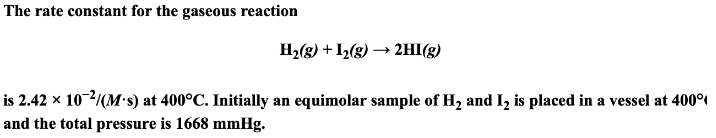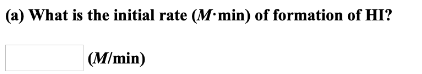# To rate constant for the gaseous reaction H2(g) + I2(g) → 2HI(g) is 2.42 x 10^-2/(M.s) at 400 degrees C. Initially an equimolar sample of H2 and I2 is placed in a vessel at 400 degrees C and the total pressure is 1668 mmHg. a) What is the initial rate (M.min) of formation of HI?